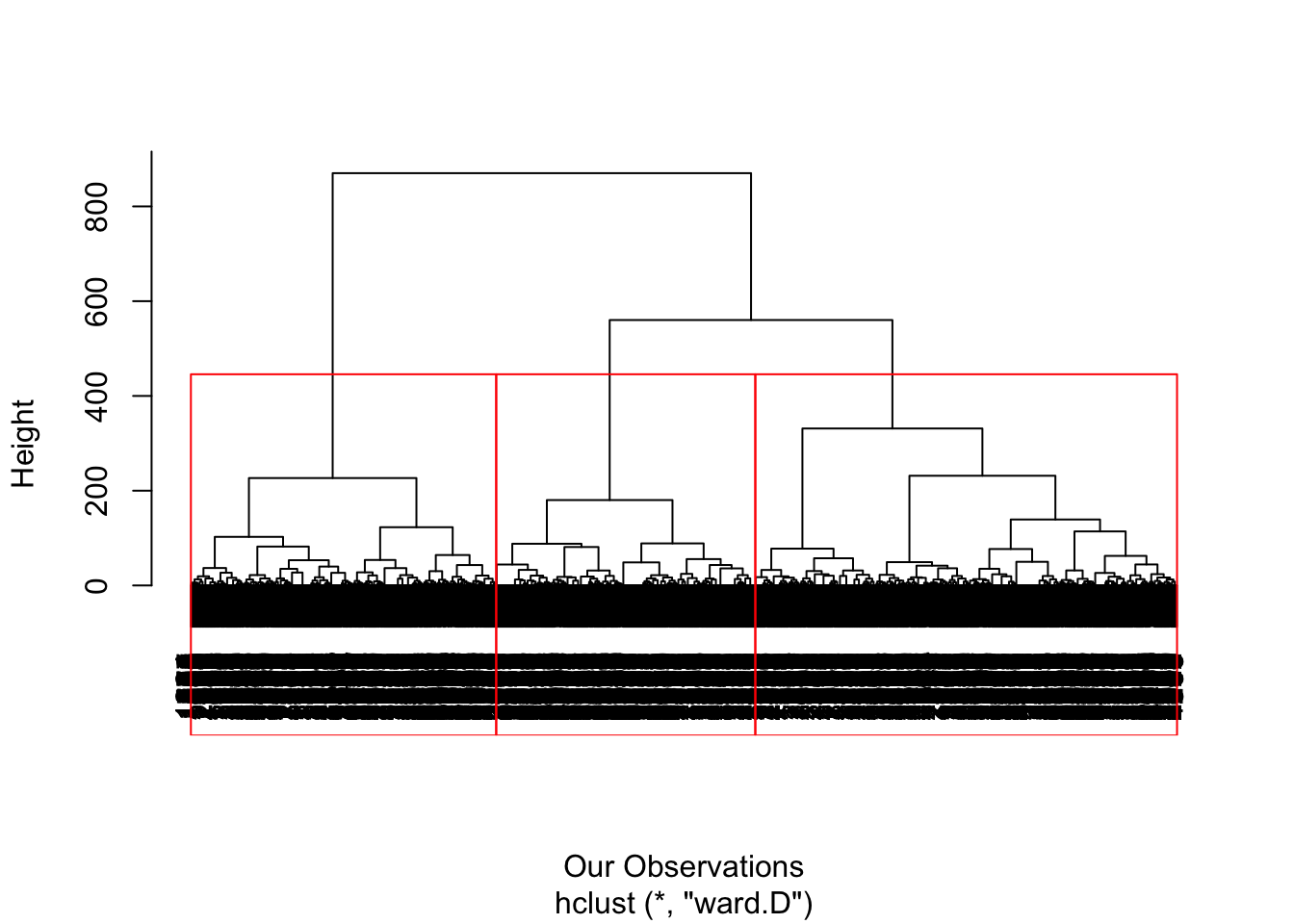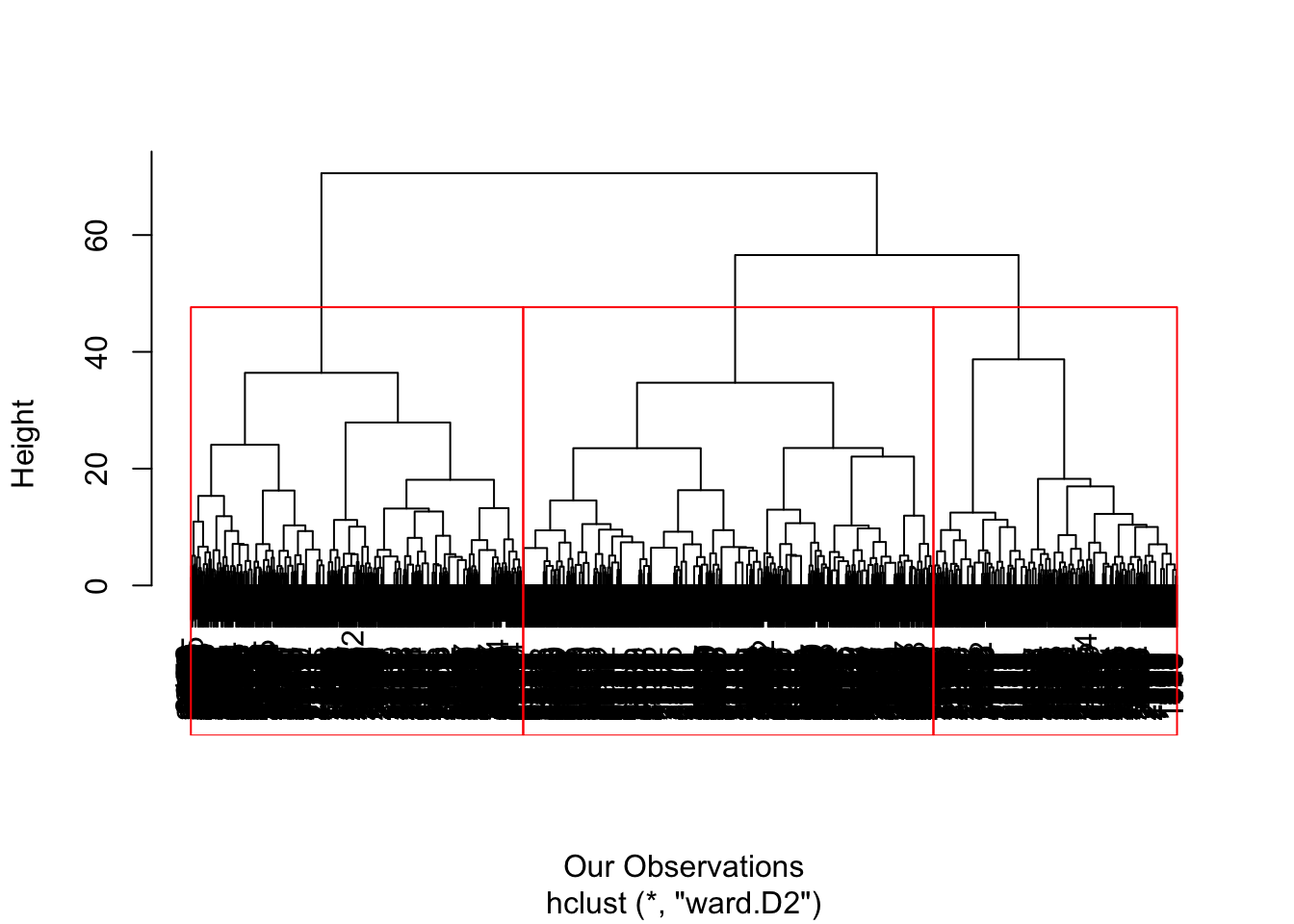Here is how you can create a very long document using only very few lines of code.

Let’s consider the boats case study as an example. Let’s only consider the cluster analysis part of the case solution. Here is how to get a report with all possible number of hierarchical cluster analysis methods tested (and endlessly more if needed). Of course one needs to then see all this output to decide what method is the best (statistically, interpretation, business-wise). Adding also all k-means methods (or other methods) will just make the report longer, but your write up time will not change much!

Let’s first run all necessary code from the analysis.

Check this first (manually), as always.

``````getwd()
setwd("CourseSessions/Sessions45")
list.files()
rm(list = ls())  # Clean up the memory, if we want to rerun from scratch``````

The focus in this example is on the hierarchical clustering segmentation part only (this is an example anyway), so we assume we selected the factors already (of course one can also change the number of factors used, all automatically).

``````ProjectData <- read.csv("data/Boats.csv", sep = ";", dec = ",")  # this contains only the matrix ProjectData
ProjectData = data.matrix(ProjectData)
colnames(ProjectData) <- gsub("\\.", " ", colnames(ProjectData))
ProjectDataFactor = ProjectData[, c(2:30)]

segmentation_attributes_used = c(10, 19, 5, 12, 3)
profile_attributes_used = 2:ncol(ProjectData)
ProjectData_segment = ProjectData[, segmentation_attributes_used]
ProjectData_profile = ProjectData[, profile_attributes_used]``````

And now we just need to call this new function `repetitioncode_example` defined in file `repetitioncode_example.R` using all possible variations of inputs (e.g. about the `distance_used` or the `hclust_method` in this example).

``````library(pryr) # make sure you installed this one
source("repetitioncode_example.R") # see what this does. all the trick is there and in the use of "results='asis'" for this code chunk
numb_clusters_used = 3  # let's not generate 100 pages!

#for (distance_used in c("euclidean","maximum")) # see help(dist), add any of these (but the more you add the longer the report will be) c("euclidean", "maximum", "manhattan", "canberra", "binary","minkowski")
#  for (hclust_method in c("ward.D","ward.D2")) { # see help(hclust), add any of these (but the more you add the longer the report will be)  c("ward.D","ward.D2", "single", "complete", "average", "mcquitty", "median", "centroid")

for (distance_used in c("euclidean", "maximum", "manhattan", "canberra", "binary","minkowski")) # see help(dist), add any of these (but the more you add the longer the report will be) c("euclidean", "maximum", "manhattan", "canberra", "binary","minkowski")
for (hclust_method in c("ward.D","ward.D2", "single", "complete", "average", "mcquitty", "median", "centroid")) { # see help(hclust), add any of these (but the more you add the longer the report will be)  c("ward.D","ward.D2", "single", "complete", "average", "mcquitty", "median", "centroid")

tmp = repetitioncode_example(distance_used,hclust_method,numb_clusters_used,ProjectData_segment)
cat("<br><hr><br>")
cat(tmp\$text1)
cat("<br>")
tmp\$plot1
cat("<br>")
cat(tmp\$text2)
cat("<br>")
print(tmp\$Line,'chart')
cat("<br>")
cat(tmp\$text3)
cat("<br> <br>")
}``````We now use as distance_used the method euclidean and as hclust_method used the method ward.D

Finally, we can see the dendrogram (see class readings and online resources for more information) to have a first rough idea of what segments (clusters) we may have - and how many.

We can also plot the ‘distances’ traveled before we need to merge any of the lower and smaller in size clusters into larger ones - the heights of the tree branches that link the clusters as we traverse the tree from its leaves to its root. If we have n observations, this plot has n-1 numbers.We now use as distance_used the method euclidean and as hclust_method used the method ward.D2

Finally, we can see the dendrogram (see class readings and online resources for more information) to have a first rough idea of what segments (clusters) we may have - and how many.

We can also plot the ‘distances’ traveled before we need to merge any of the lower and smaller in size clusters into larger ones - the heights of the tree branches that link the clusters as we traverse the tree from its leaves to its root. If we have n observations, this plot has n-1 numbers.